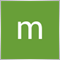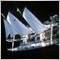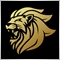# What is wrong in my code? Getting error 138240

```int Close1B()
{

int      Close1B=-1;                  // None open.
for(int cnt=OrdersTotal()-1;cnt>=0;cnt--)
{
//if( ! OrderSelect(cnt, SELECT_BY_POS, MODE_TRADES) ) continue;
if(OrderSymbol()==Symbol()
&& (OrderType()==OP_BUY) && (OrderLots()==Order0_Lot) && (OrderComment()=="First"))

Close1B=OrderTicket();
RefreshRates();

}
//----
return(Close1B);
}
//+------------------------------------------------------------------+
//|                                                                  |
//+------------------------------------------------------------------+
int Close2B()
{

int      Close2B=-1;                  // None open.
for(int cnt=OrdersTotal()-1;cnt>=0;cnt--)
{
//if( ! OrderSelect(cnt, SELECT_BY_POS, MODE_TRADES) ) continue;
if(OrderSymbol()==Symbol()
&& (OrderType()==OP_BUY) && (OrderLots()==Order1_Lot) && (OrderComment()=="M1"))

Close2B=OrderTicket();
RefreshRates();

}
//----
return(Close2B);
}
//+------------------------------------------------------------------+
//|                                                                  |
//+------------------------------------------------------------------+
int Close3B()
{

int      Close3B=-1;                  // None open.
for(int cnt=OrdersTotal()-1;cnt>=0;cnt--)
{
//if( ! OrderSelect(cnt, SELECT_BY_POS, MODE_TRADES) ) continue;
if(OrderSymbol()==Symbol()
&& (OrderType()==OP_BUY) && (OrderLots()==Order2_Lot) && (OrderComment()=="M2"))

Close3B=OrderTicket();
RefreshRates();

}
//----
return(Close3B);
}
//+------------------------------------------------------------------+
//|                                                                  |
//+------------------------------------------------------------------+
int Close4B()
{

int      Close4B=-1;                  // None open.
for(int cnt=OrdersTotal()-1;cnt>=0;cnt--)
{
//if( ! OrderSelect(cnt, SELECT_BY_POS, MODE_TRADES) ) continue;
if(OrderSymbol()==Symbol()
&& (OrderType()==OP_BUY) && (OrderLots()==Order3_Lot) && (OrderComment()=="M3"))

Close4B=OrderTicket();
RefreshRates();

}
//----
return(Close4B);
}
//+------------------------------------------------------------------+
//|                                                                  |
//+------------------------------------------------------------------+
int Close5B()
{

int      Close5B=-1;                  // None open.
for(int cnt=OrdersTotal()-1;cnt>=0;cnt--)
{
//if( ! OrderSelect(cnt, SELECT_BY_POS, MODE_TRADES) ) continue;
if(OrderSymbol()==Symbol()
&& (OrderType()==OP_BUY) && (OrderLots()==Order4_Lot) && (OrderComment()=="M4"))

Close5B=OrderTicket();
RefreshRates();

}
//----
return(Close5B);
}
//+------------------------------------------------------------------+
//|                                                                  |
//+------------------------------------------------------------------+
int Close6B()
{

int      Close6B=-1;                  // None open.
for(int cnt=OrdersTotal()-1;cnt>=0;cnt--)
{
//if( ! OrderSelect(cnt, SELECT_BY_POS, MODE_TRADES) ) continue;
if(OrderSymbol()==Symbol()
&& (OrderType()==OP_BUY) && (OrderLots()==Order5_Lot) && (OrderComment()=="M5"))

Close6B=OrderTicket();
RefreshRates();

}
//----
return(Close6B);
}
//+------------------------------------------------------------------+
//|                                                                  |
//+------------------------------------------------------------------+
int Close1S()
{

int      Close1S=-1;                  // None open.
for(int cnt=OrdersTotal()-1;cnt>=0;cnt--)
{
//if( ! OrderSelect(cnt, SELECT_BY_POS, MODE_TRADES) ) continue;
if(OrderSymbol()==Symbol()
&& (OrderType()==OP_SELL) && (OrderLots()==Order0_Lot) && (OrderComment()=="First"))

Close1S=OrderTicket();
RefreshRates();

}
//----
return(Close1S);
}
//+------------------------------------------------------------------+
//|                                                                  |
//+------------------------------------------------------------------+
int Close2S()
{

int      Close2S=-1;                  // None open.
for(int cnt=OrdersTotal()-1;cnt>=0;cnt--)
{
//if( ! OrderSelect(cnt, SELECT_BY_POS, MODE_TRADES) ) continue;
if(OrderSymbol()==Symbol()
&& (OrderType()==OP_SELL) && (OrderLots()==Order1_Lot) && (OrderComment()=="M1"))

Close2S=OrderTicket();
RefreshRates();

}
//----
return(Close2S);
}
//+------------------------------------------------------------------+
//|                                                                  |
//+------------------------------------------------------------------+
int Close3S()
{

int      Close3S=-1;                  // None open.
for(int cnt=OrdersTotal()-1;cnt>=0;cnt--)
{
//if( ! OrderSelect(cnt, SELECT_BY_POS, MODE_TRADES) ) continue;
if(OrderSymbol()==Symbol()
&& (OrderType()==OP_SELL) && (OrderLots()==Order2_Lot) && (OrderComment()=="M2"))

Close3S=OrderTicket();
RefreshRates();

}
//----
return(Close3S);
}
//+------------------------------------------------------------------+
//|                                                                  |
//+------------------------------------------------------------------+
int Close4S()
{

int      Close4S=-1;                  // None open.
for(int cnt=OrdersTotal()-1;cnt>=0;cnt--)
{
//if( ! OrderSelect(cnt, SELECT_BY_POS, MODE_TRADES) ) continue;
if(OrderSymbol()==Symbol()
&& (OrderType()==OP_SELL) && (OrderLots()==Order3_Lot) && (OrderComment()=="M3"))

Close4S=OrderTicket();
RefreshRates();

}
//----
return(Close4S);
}
//+------------------------------------------------------------------+
//|                                                                  |
//+------------------------------------------------------------------+
int Close5S()
{

int      Close5S=-1;                  // None open.
for(int cnt=OrdersTotal()-1;cnt>=0;cnt--)
{
//if( ! OrderSelect(cnt, SELECT_BY_POS, MODE_TRADES) ) continue;
if(OrderSymbol()==Symbol()
&& (OrderType()==OP_SELL) && (OrderLots()==Order4_Lot) && (OrderComment()=="M4"))

Close5S=OrderTicket();
RefreshRates();

}
//----
return(Close5S);
}
//+------------------------------------------------------------------+
//|                                                                  |
//+------------------------------------------------------------------+
int Close6S()
{

int      Close6S=-1;                  // None open.
for(int cnt=OrdersTotal()-1;cnt>=0;cnt--)
{
//if( ! OrderSelect(cnt, SELECT_BY_POS, MODE_TRADES) ) continue;
if(OrderSymbol()==Symbol()
&& (OrderType()==OP_SELL) && (OrderLots()==Order5_Lot) && (OrderComment()=="M5"))

Close6S=OrderTicket();
RefreshRates();

}
//----
return(Close6S);
}
//+------------------------------------------------------------------+
//|                                                                  |
//+------------------------------------------------------------------+
{ //start

{
// do what you want.

OrderClose(Close1B(),Order0_Lot,MarketInfo(OrderSymbol(),MODE_BID),2,Red);

}
{
// do what you want.
OrderClose(Close2B(),Order1_Lot,MarketInfo(OrderSymbol(),MODE_BID),2,Red);
}
{
// do what you want.
OrderClose(Close3B(),Order2_Lot,MarketInfo(OrderSymbol(),MODE_BID),2,Red);
}
{
// do what you want.
OrderClose(Close4B(),Order3_Lot,MarketInfo(OrderSymbol(),MODE_BID),2,Red);
}

{
// do what you want.
OrderClose(Close5B(),Order4_Lot,MarketInfo(OrderSymbol(),MODE_BID),2,Red);
}

if((Next_SELL_Lot_Size==Order1_Lot) && (OrderType()==OP_SELL)) // compare the commenting
{
// do what you want.

}
if((Next_SELL_Lot_Size==Order2_Lot) && (OrderType()==OP_SELL)) // compare the commenting
{
// do what you want.
}
if((Next_SELL_Lot_Size==Order3_Lot) && (OrderType()==OP_SELL)) // compare the commenting
{
// do what you want.
}
if((Next_SELL_Lot_Size==Order4_Lot) && (OrderType()==OP_SELL)) // compare the commenting
{
// do what you want.
}

if((Next_SELL_Lot_Size==Order5_Lot) && (OrderType()==OP_SELL)) // compare the commenting
{
// do what you want.

}

//end
}```
I run my function in the onTimer event.38646

mql4 topic in mql4 section of the forum please.Moderator
20313

What is error 138?533

Marth Nataka

With respect... you should rather ask someone that question when you are lying down on a long comfortable couch... You should not be programming if you need to ask that question....

But I'm interested... what are you trying to do exactly...?240

MikeT:

Marth Nataka

With respect... you should rather ask someone that question when you are lying down on a long comfortable couch... You should not be programming if you need to ask that question....

But I'm interested... what are you trying to do exactly...?

Close the last trade at the open of the next240

Keith Watford:
What is error 138?
 138 ERR_REQUOTE RequoteModerator
12666

The requested price is not or no longer valid or the bid and ask are mixed up.Moderator
20313

Keith Watford:
What is error 138?
marth tanaka:
 138 ERR_REQUOTE Requote

Please don't just post the error code, also add the error description.

People are less likely to help you if they have to look up the description themselves.240

Keith Watford:

Please don't just post the error code, also add the error description.

People are less likely to help you if they have to look up the description themselves.

EURUSD,M1: OrderClose error 138240

Marco vd Heijden:
The requested price is not or no longer valid or the bid and ask are mixed up.
hmmm,

I know for sure the bid and ask prices arent mixed up. Im grabbing the requested price using MarketInfo(OrderSymbol(),MODE_BID) and MarketInfo(OrderSymbol(),MODE_ASK) so I dont know why I am still getting this problem. The problem certainly lies within the TradeCloser function() since I get the error
EURUSD,M1: OrderClose error 138Moderator
12666

It can not be the TradeCloser() function because it is nowhere called.

Are you sure you have correctly selected the order you want to close ?

For example you can not call OrderSymbol() or OrderType() when you don't have an order selected .